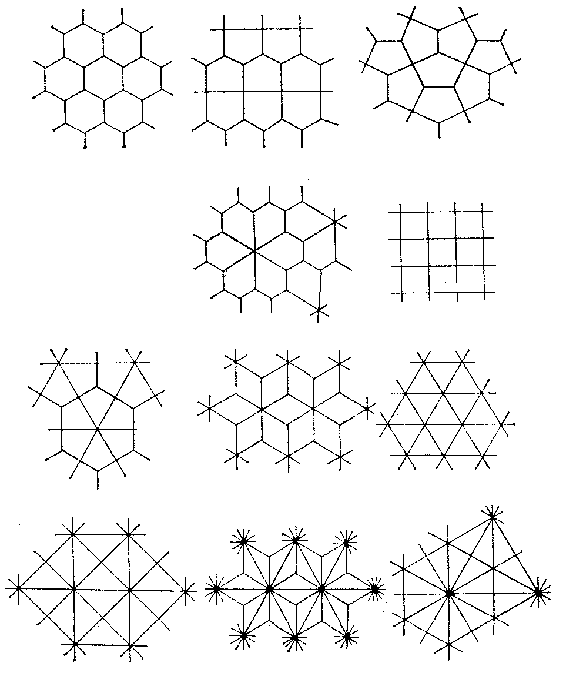# PlanigonThe planigons in the Lobachevskii plane are regular polygons with an arbitrary number of sides $k$ such that an arbitrary fixed number $\alpha$ of them meet at each vertex of the planigon. For numbers of sides $k=3,4,5,6$, and $>6$, one may choose a planigon such that $\alpha\geq7$, $\geq5$, $\geq4$, $\geq4$, and $\geq3$. The multi-dimensional analogue of a planigon is a stereohedron.